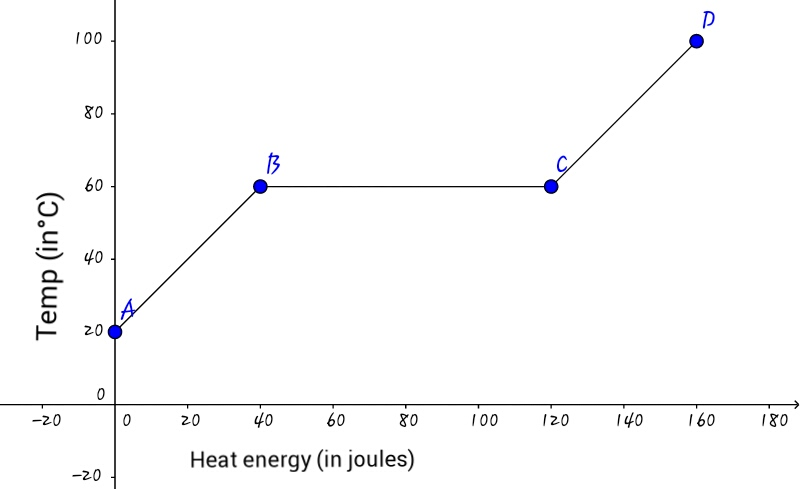# An AC would surely help!

A solid initially at $20\ ^\circ\text{C}$ is heated. The graph shows variation in temperature with the amount of heat energy supplied to it. Now, if the specific heat capacity of the solid is $2\text{ J}{g}^{-1}\ ^\circ\text{C}^{-1},$ calculate

(i) the mass of the solid and
(ii) the specific latent heat of fusion of the solid.

Submit your answer as the sum of (i) (in grams) and (ii) $($in $\text{ J/g}).$×